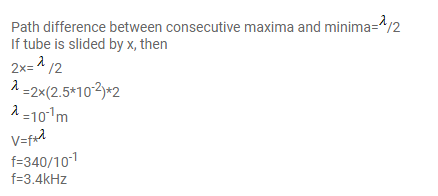# In Quincke's experiment the sound detected is changed`
Question:

In Quincke's experiment the sound detected is changed from a maximum to a minimum when the sliding tube is moved through a distance of $2.50 \mathrm{~cm}$. Find the frequency of sound if the speed of sound in air is $340 \mathrm{~m} \mathrm{~s}^{-1}$.

Solution: